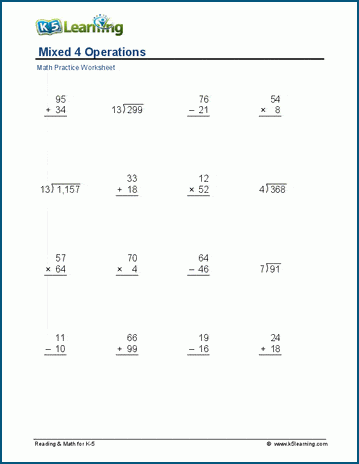# Multi-digit Math Drill Practice

These worksheets cover 2-, 3- and 4-digit addition, subtraction, multiplication, and division math drills. Let us show you some of the worksheets.

## Mixed addition and subtraction practice

This section includes a series of worksheets that cover 2-digit, 3-digit and 4-digit addition and subtraction math drills. Each section has pages with 25 questions and with 48 questions, depending on how many you want your child to work on.

The below worksheet covers 3-digit addition and subtraction, and it displays 25 questions.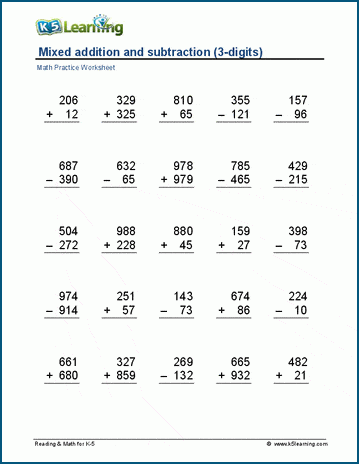## Mixed multiplication and division worksheets

We have two separate sets of math drill worksheets for mixed 2-digit and 3-digit multiplication and division.

The below worksheet covers 3-digit multiplication and division.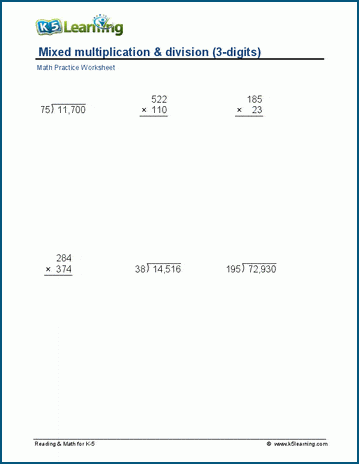## Missing factors practice

These sets of 1x2 digit and larger numbers worksheets cover missing factors.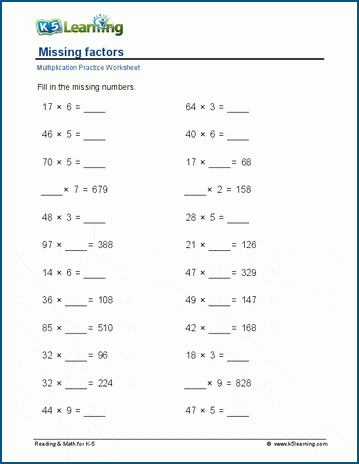## Missing divisor and dividends worksheets

The next set pf worksheets have division questions with missing questions. The first set covers 1-digit divisors, and the next set larger numbers.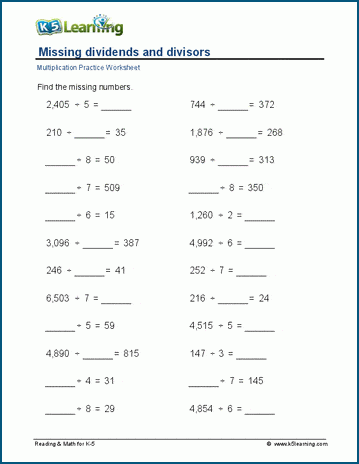## Mixed 4 operations practice

The final two sets of worksheets cover all four operations mixed practice. The first set covers 1 – 3 digits and the second set 2 – 4 digits.

The below worksheet covers 1 – 3 digits.